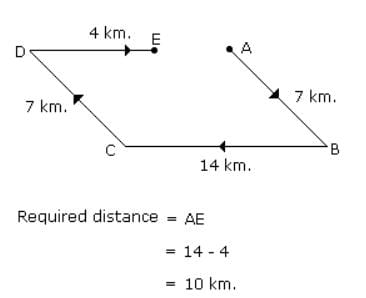Test: Rest And Motion

# Test: Rest And Motion - Class 9

Test Description

## 10 Questions MCQ Test Science Class 9 - Test: Rest And Motion

Test: Rest And Motion for Class 9 2023 is part of Science Class 9 preparation. The Test: Rest And Motion questions and answers have been prepared according to the Class 9 exam syllabus.The Test: Rest And Motion MCQs are made for Class 9 2023 Exam. Find important definitions, questions, notes, meanings, examples, exercises, MCQs and online tests for Test: Rest And Motion below.
Solutions of Test: Rest And Motion questions in English are available as part of our Science Class 9 for Class 9 & Test: Rest And Motion solutions in Hindi for Science Class 9 course. Download more important topics, notes, lectures and mock test series for Class 9 Exam by signing up for free. Attempt Test: Rest And Motion | 10 questions in 10 minutes | Mock test for Class 9 preparation | Free important questions MCQ to study Science Class 9 for Class 9 Exam | Download free PDF with solutions
 1 Crore+ students have signed up on EduRev. Have you?
Test: Rest And Motion - Question 1

### Rest state and motion of body are

Detailed Solution for Test: Rest And Motion - Question 1

Rest and motion are the relative terms because they depend on the observer’s frame of reference.
If an observer is at rest in his or her own frame of reference, but he may be moving in other observer's frame of reference. So if two different observers are not at rest with respect to each other, then they too get different results when they observe the motion or rest of a body.

Test: Rest And Motion - Question 2

### An ant moves from one corner of a hall to the diagonally opposite corner. If the dimension of the hall are 8 m x 6 m, the displacement of the ant is :

Detailed Solution for Test: Rest And Motion - Question 2

In this case
Displacement = hypotenuse
Displacement = (82 +62)1/2 = 10 m

Test: Rest And Motion - Question 3

### The displacement covered by a seconds’ hand of radius r in a clock after one revolution is:

Detailed Solution for Test: Rest And Motion - Question 3

"Displacement will be zero because displacement is the difference between initial and final position but after one revolution the second hand came to its initial position; so it's final and initial position is same so displacement is zero "

Test: Rest And Motion - Question 4

A man leaves his house at 7:30 a.m. for a morning walk, and returns back at 8:15 a.m. after covering 3 km. Is displacement in this time is:

Detailed Solution for Test: Rest And Motion - Question 4

His displacement will be zero although distance covered is non-zero since he ends up where he started.

Displacement can be zero but distance cannot be zero because distance is the total path covered but displacement is the shortest distance between the initial and final position. It can be zero at the end of one round.

Test: Rest And Motion - Question 5

A body travels from A to B at 40m/s and from B to A at 60m/s. Calculate the average speed.

Detailed Solution for Test: Rest And Motion - Question 5

Let n be the distance between a and b. Then, the total time taken would be:

n/40 + n/60

And the total distance is 2n. So:

V=distance/time=2n/ n/40 + n/60

=240n/3n+2n

=240/5

=48 m/s

Test: Rest And Motion - Question 6

A car moved 60 km East and 90 km West. What is the distance?

Detailed Solution for Test: Rest And Motion - Question 6

60km.east + 90km.west =150km.

Test: Rest And Motion - Question 7

A body is said to be in non-uniform motion if it travels

Detailed Solution for Test: Rest And Motion - Question 7

Non Uniform motion is defined as the motion of an object in which the object travels with varied speed and it does not cover same distance in equal time intervals, irrespective of the time interval length.

Examples:

1. If a car covers 10 meters in first two seconds, and 15 meters in next two seconds.

2. The motion of a train.

Test: Rest And Motion - Question 8

A Quantity which has both magnitude and direction is called.

Detailed Solution for Test: Rest And Motion - Question 8

Vector quantity has both magnitude and direction but scalar quantity have only magnitude

Test: Rest And Motion - Question 9

If a body does not changes its position with respect to its surroundings, it is said to be at

Detailed Solution for Test: Rest And Motion - Question 9

A body whose position with respect to surrounding doesn't change is said to be in a state of rest .

Test: Rest And Motion - Question 10

Radha moves towards South-East a distance of 7 km, then she moves towards West and travels a distance of 14 km. From here she moves towards North-West a distance of 7 km and finally she moves a distance of 4 km towards east. How far is she now from the starting point?

Detailed Solution for Test: Rest And Motion - Question 10## Science Class 9

66 videos|355 docs|97 tests
Information about Test: Rest And Motion Page
In this test you can find the Exam questions for Test: Rest And Motion solved & explained in the simplest way possible. Besides giving Questions and answers for Test: Rest And Motion, EduRev gives you an ample number of Online tests for practice

## Science Class 9

66 videos|355 docs|97 tests